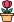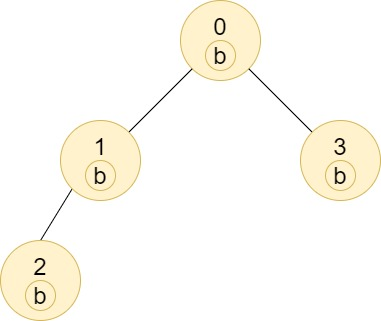엔지니어 게시판
LeetCode 솔루션 분류

# [1/12] 1519. Number of Nodes in the Sub-Tree With the Same Label

### 작성자 정보

•작성
• 작성일

• 111 조회
• 1 댓글
• 0 추천
• 0 비추천

### 본문

1519. Number of Nodes in the Sub-Tree With the Same Label

You are given a tree (i.e. a connected, undirected graph that has no cycles) consisting of `n` nodes numbered from `0` to `n - 1` and exactly `n - 1` `edges`. The root of the tree is the node `0`, and each node of the tree has a label which is a lower-case character given in the string `labels` (i.e. The node with the number `i` has the label `labels[i]`).

The `edges` array is given on the form `edges[i] = [ai, bi]`, which means there is an edge between nodes `ai` and `bi` in the tree.

Return an array of size `n` where `ans[i]` is the number of nodes in the subtree of the `ith` node which have the same label as node `i`.

A subtree of a tree `T` is the tree consisting of a node in `T` and all of its descendant nodes.

Example 1:```Input: n = 7, edges = [[0,1],[0,2],[1,4],[1,5],[2,3],[2,6]], labels = "abaedcd"
Output: [2,1,1,1,1,1,1]
Explanation: Node 0 has label 'a' and its sub-tree has node 2 with label 'a' as well, thus the answer is 2. Notice that any node is part of its sub-tree.
Node 1 has a label 'b'. The sub-tree of node 1 contains nodes 1,4 and 5, as nodes 4 and 5 have different labels than node 1, the answer is just 1 (the node itself).
```

Example 2:```Input: n = 4, edges = [[0,1],[1,2],[0,3]], labels = "bbbb"
Output: [4,2,1,1]
Explanation: The sub-tree of node 2 contains only node 2, so the answer is 1.
The sub-tree of node 3 contains only node 3, so the answer is 1.
The sub-tree of node 1 contains nodes 1 and 2, both have label 'b', thus the answer is 2.
The sub-tree of node 0 contains nodes 0, 1, 2 and 3, all with label 'b', thus the answer is 4.
```

Example 3:```Input: n = 5, edges = [[0,1],[0,2],[1,3],[0,4]], labels = "aabab"
Output: [3,2,1,1,1]
```

Constraints:

• `1 <= n <= 105`
• `edges.length == n - 1`
• `edges[i].length == 2`
• `0 <= ai, bi < n`
• `ai != bi`
• `labels.length == n`
• `labels` is consisting of only of lowercase English letters.
Accepted
75.3K
Submissions
135.6K
Acceptance Rate
<div class="text-label-1 dark:text-dark-label-1 text-sm font-medium" style="border-width: 0px; border-style: solid; border-color: var(--chakra-colors-chakra-border-color); border-image: initial; box-sizing: border-box; --tw-border-spacing-x:0; --tw-border-spacing-y:0; --tw-translate-x:0; --tw-translate-y:0; --tw-rotate:0; --tw-skew-x:0; --tw-skew-y:0; --tw-scale-x:1; --tw-scale-y:1; --tw-pan-x: ; --tw-pan-y: ; --tw-pinch-zoom: ; --tw-scroll-snap-strictness:proximity; --tw-ordinal:

댓글 1

## 학부유학생님의 댓글

• 익명
• 작성일
``````from collections import Counter, defaultdict
class Solution:
def countSubTrees(self, n: int, edges: List[List[int]], labels: str) -> List[int]:
graph = defaultdict(list)
for e1, e2 in edges:
graph[e1].append(e2)
graph[e2].append(e1)

res = *n

def dfs(prev, curr):

counter = Counter()
for nxt in graph[curr]:
if nxt == prev: continue
counter = counter + dfs(curr, nxt)
counter[labels[curr]] += 1
res[curr] = counter[labels[curr]]

return counter

dfs(-1, 0)
return res``````
전체 349 / 1 페이지

• 등록일 00:48
• 등록일 02.01
• 등록일 02.01
• 등록일 02.01
• 등록일 02.01
• 등록일 02.01
• 등록일 02.01
• 등록일 02.01
• 등록일 02.01
• 등록일 02.01
• 등록일 02.01
• 등록일 02.01

• 등록일 01.31
• 등록일 01.31
• 등록일 01.31
• 등록일 01.27
• 등록일 01.27
• 등록일 01.23
• 등록일 01.23